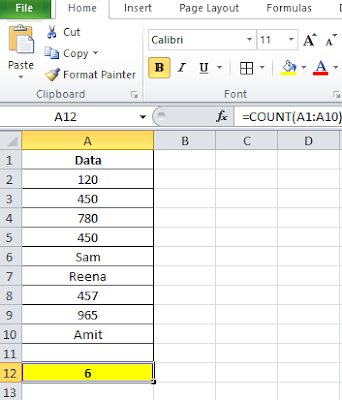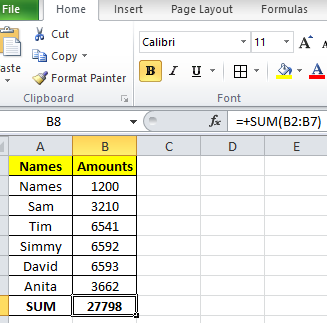Advanced excel has a simple meaning of all features and functions of Microsoft Excel is used by users to handle large calculations and data processing. Large data analysing and solving big issues related to any data in Excel. Microsoft Excel is a champion among the least demanding and weighty programming applications open out there.Advanced Excel

Now we will discuss advanced excel in detail but before proceeding let s understand what is basic excel also.

Basic excel consists of some of the basic functions or formulas in excel Now we used the word basic so it does not mean that basic excel is nothing or not needed to learn. Basic excel has also an important role because if we do not understand basic steps or functions in excel then, of course, we will have an issue understanding advanced excel.

## Basic Excel

Now as we said basic excel is also important to learn so here we will add some basic functions first to understand.

Basic functions consist of those functions that we can see below. Let us have a look here.

Sum: This function is very nice as we can use it to add the numbers as a sum means we can add any columns or rows that consist of numbers.

Count: We can use this function easily to count the cells or rows if there is data in cells.

For example.

If A1: A10 =Count(A1: A10), If we mention any numbers in cells, would be counted except text in cells. This means if we mention numbers in cells those would be counted but if we mention texts in cells they will not be counted. It gives a final result of the count function based on numbers in cells.Count Function
This is called the COUNT function in excel.

Average: This function counts the cells and calculates the average of the total cells that consists of numbers not texts, Let us take an example here.

If we mention any numbers in cells in rows or columns like this below.

A1: A10 = Average(A1: A10)

This function will only calculate the cells where numbers are mentioned in the data and if we mentioned some texts in there then the text will be ignored.Average Function

Now we have seen that in the image above all data has been calculated as per the Average function and the outcome is 537 finally which is an average of the total data that we have in the above cells in excel. Also, we can see texts in some cells which were ignored by this average function.

Though there are many basic functions in excel which are very important to learn before we go to an advanced level in excel. Now here we will discuss advanced excel with formulas and examples.

1. Pivot Table & Reporting
2. Pivot Charts
3. VBA & Macros
4. Conditional Formatting
5. Data Validation
1. Creating a Dashboard in Excel
5. Data Tables
1. Tables & Formatting
2. Index+Match
3. Formula Auditing
4. Array Formulas
5. Sumifs
When we talk about Advanced Excel then we must say that if anybody knows about advanced excel then he or she feels very easy to handle any kind of data processing. Advanced excel simply makes people smart because when you learn it and you can crunch the data smartly.

If we talk about even one of the advanced excel functions which is Conditional Formatting gives an easy solution to work as per any data conditions. With help of this feature, data can be highlighted with conditions needed in excel.

If Function
Meaning of IF Instruction: According to Microsoft Excel, IF articulation is characterized as a capacity that "checks whether a condition is met, returns one esteem assuming True and another esteem assuming False".Advanced Excel- IF function

The best other feature which is mentioned above is PIVOT-TABLE which is being used in Ms excel to acknowledge any huge data and convert that into a summarizing way which will be an easy way to check and acknowledge.

Now when we talk about PIVOT-CHART so, of course, we should know about Pivot-Table as Pivot-Chart will be used after putting the Pivot-Table function in the Excel sheet.

When this Pivot-table is ready then we can simply create a Pivot-Chart by using an INSERT tab and create a Pivot-Chart as it is there in the drop-down option OR When you will click anywhere in the chart then it will easily appear as a chart ribbon.

Data Tables – It helps users to analyse a massive amount of data for a solution.

Vlookup helps users to find any particular value or text in any data Vertically. Which is a very usual and important function nowadays. Even MIS users also need it so they learn it and go with advanced lookup too then.

When we use Vlookup with multiple conditions in excel, Called advanced Vlookup. Nothing is different as it is just a way of handling huge data.

HLOOKUP in EXCEL:- It helps users to find the values Horizontally and Advanced Hlookup is handling huge data with multiple conditions in excel.

When we want to add numbers in the range like starting from 01 to 150 or any number with one criterion, Called Sumif in excel. When we add multiple criteria then it is called the SUMIFS function in excel.

Data Validation is also one of the best advanced excel functions as it helps users to put conditions in data like as per numbers or texts. For example:- If we need numbers from 01 to 250 then we can put this condition so that numbers will appear above 250 in the column or row accordingly.

Question:- How to use the Sum function in excel called a basic feature of ms excel?
Answer:- The syntax is as follows:

### =SUM(B2: B7)Advanced Excel -  Sum Function

Now Dashboard in excel is something the most important and interesting functions also in Ms excel. It gives a complete solution to summarize the data in a basic and dynamic way. It would be created with Pivot-Table and then can be converted into charts or basic Tables in excel.